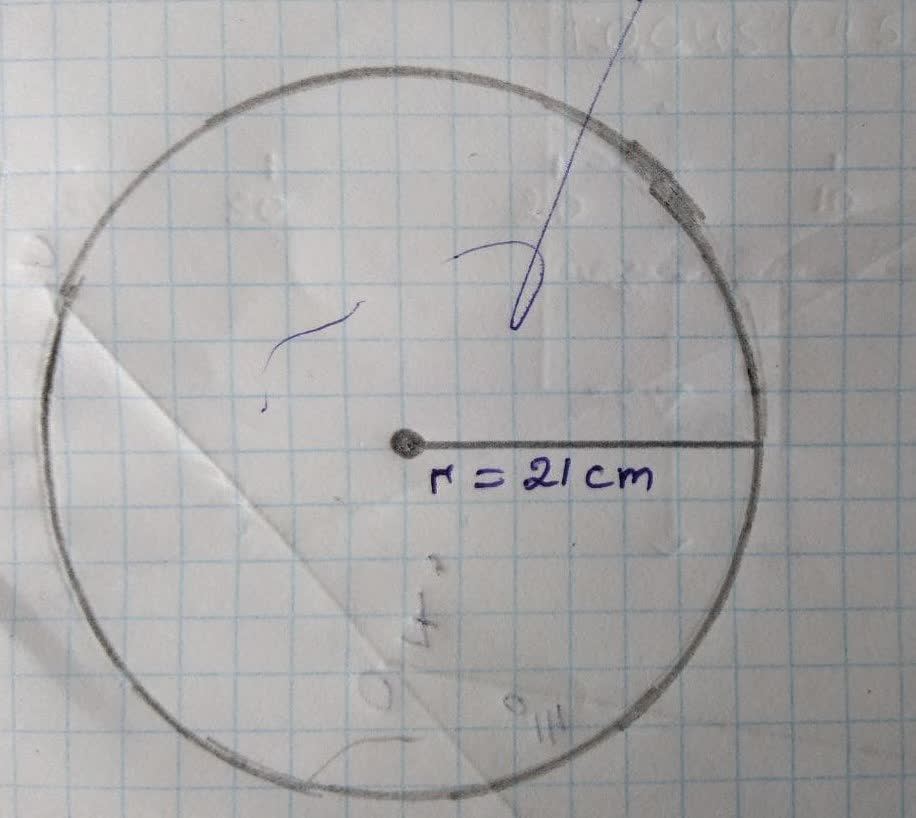# What is the circumference of the circle? Use \frac{22}{7} forjippie771h 2021-11-29 Answered
What is the circumference of the circle? Use $\frac{22}{7}$ for x.
a) 44 cm
b) 66 cm
c) 182 cm
d) 154 cm
You can still ask an expert for help

• Questions are typically answered in as fast as 30 minutes

Solve your problem for the price of one coffee

• Math expert for every subject
• Pay only if we can solve itJick1984

Step1
Given:
Similarto circle sphere is a two dimensional space where the set of points that are at the same distance r from agiven point in a three dimensional space. In analytical geometry with a center and radius is the locus of all points is called sphere
Step2
Given:Step3
Circumference
The distance around a circle is called its circumference.
The circumference of the circle is $C=2\pi r$
Taking $\pi \approx \frac{22}{7}$
Step4
The object is to find the circumference for the given circle as follows,
The radius of the circle is r=21
Step5
Then the circumference of the circle is,
$C=2\pi r$
$C=2\left(\begin{array}{c}\frac{22}{7}\end{array}\right)\left(21\right)$
.
So the circumference of the circle is approximately 132 in.